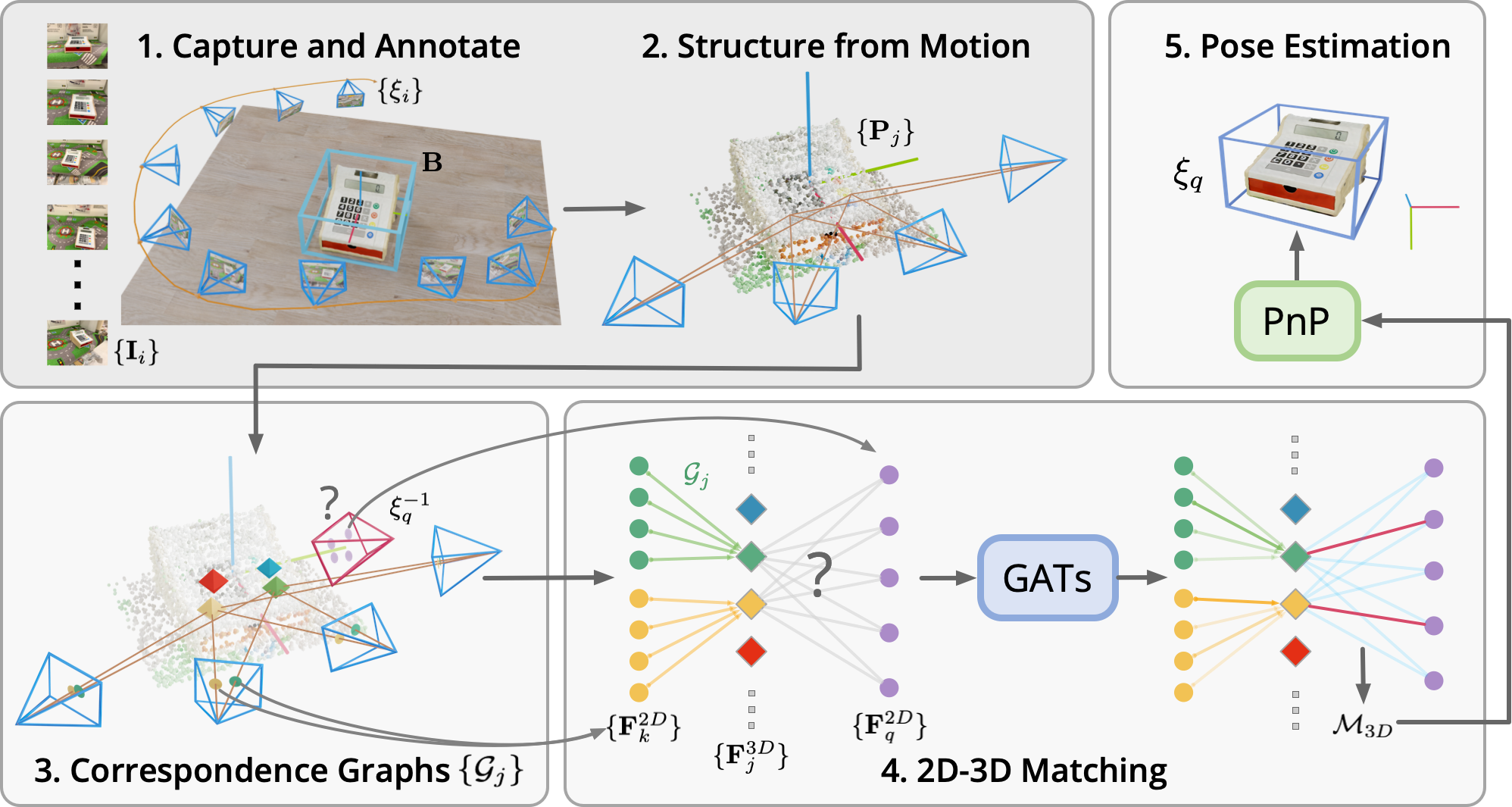## OnePose: One-Shot Object Pose Estimation without CAD Models

#### CVPR 2022

###### Jiaming Sun1,2*, Zihao Wang1*, Siyu Zhang2*, Xingyi He1, Hongcheng Zhao3, Guofeng Zhang1, Xiaowei Zhou1

1State Key Lab of CAD & CG, Zhejiang University    2SenseTime Research    3TUM
* denotes equal contribution

### Abstract

###### TL;DR: OnePose can estimate 6D poses of arbitrary household objects without instance/category-specific training or CAD models.

We propose a new method named OnePose for object pose estimation. Unlike existing instance-level or category-level methods, OnePose does not rely on CAD models and can handle objects in arbitrary categories without instance- or category-specific network training. OnePose draws the idea from visual localization and only requires a simple RGB video scan of the object to build a sparse SfM model of the object. Then, this model is registered to new query images with a generic feature matching network. To mitigate the slow runtime of existing visual localization methods, we propose a new graph attention network that directly matches 2D interest points in the query image with the 3D points in the SfM model, resulting in efficient and robust pose estimation. Combined with a feature-based pose tracker, OnePose is able to stably detect and track 6D poses of everyday household objects in real-time. We also collected a large-scale dataset that consists of 450 sequences of 150 objects.

### Pipeline overview$\textbf{1.}$ For each object, a video scan with RGB frames $\{\mathbf{I}_i\}$ and camera poses $\{\xi_{i}\}$ are collected together with the annotated 3D object bounding box $\mathbf{B}$. $\textbf{2.}$ Structure from Motion (SfM) reconstructs a sparse point cloud $\{\mathbf{P}_j\}$ of the object. $\textbf{3.}$ The correspondence graphs $\{\mathcal{G}_j\}$ are built during SfM, which represent the 2D-3D correspondences in the SfM map. $\textbf{4.}$ 2D descriptors $\{\mathbf{F}_k^{2D}\}$ are aggregated to 3D descriptors $\{\mathbf{F}_j^{3D}\}$ with the aggregration-attention layer. $\{\mathbf{F}_j^{3D}\}$ are later matched with 2D descriptors from the query image $\{\mathbf{F}_q^{2D}\}$ to generate 2D-3D match predictions $\mathcal{M}_{3D}$. $\textbf{5.}$ Finally, the object pose $\xi_{q}$ is computed by solving the PnP problem with $\mathcal{M}_{3D}$.

### Citation


@article{sun2022onepose,
title={{OnePose}: One-Shot Object Pose Estimation without {CAD} Models},
author = {Sun, Jiaming and Wang, Zihao and Zhang, Siyu and He, Xingyi and Zhao, Hongcheng and Zhang, Guofeng and Zhou, Xiaowei},
journal={CVPR},
year={2022},
}